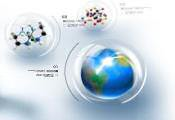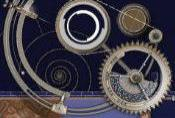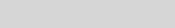Valdir Aguilera# E = m c2

Albert Einstein

It followed from the special theory of relativity that mass and energy are both but different manifestation of the same thing – a somewhat unfamiliar conception for the average mind. Furthermore, the equation E is equal to m c squared, in which energy is put equal to mass, multiplied by the square of the velocity of light, showed that very small amounts of mass may be converted into a very large amount of energy and vice versa. The mass and energy were in fact equivalent, according to the formula mentioned before. This was demonstrated by Cockcroft and Walton in 1932, experimentally.

Para ouvi-lo, clique AQUI.